ï»¿

### Introduzione

#### Abstract

En
Given a topological space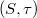$(S,\tau)$ and a subset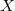$X$ of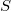$S$, the topology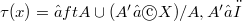$\tau(x) = â‰¤ft{A \cup (A' âˆ© X)/A,A' âˆˆ Ï„ \right}$ is called the simple extension of$\tau$ by$X$ (see Levine 3).Here we show how to recognize when two subsets$X$ and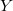$Y$ of$S$ define the same simple extension of$\tau$ and we give a characterization of those expansions of$\tau$ which are simple extensions of$\tau$ by suitable subsets of$S$.

DOI Code: §

Full Text: PDFThis work is licensed under a Creative Commons Attribuzione - Non commerciale - Non opere derivate 3.0 Italia License.